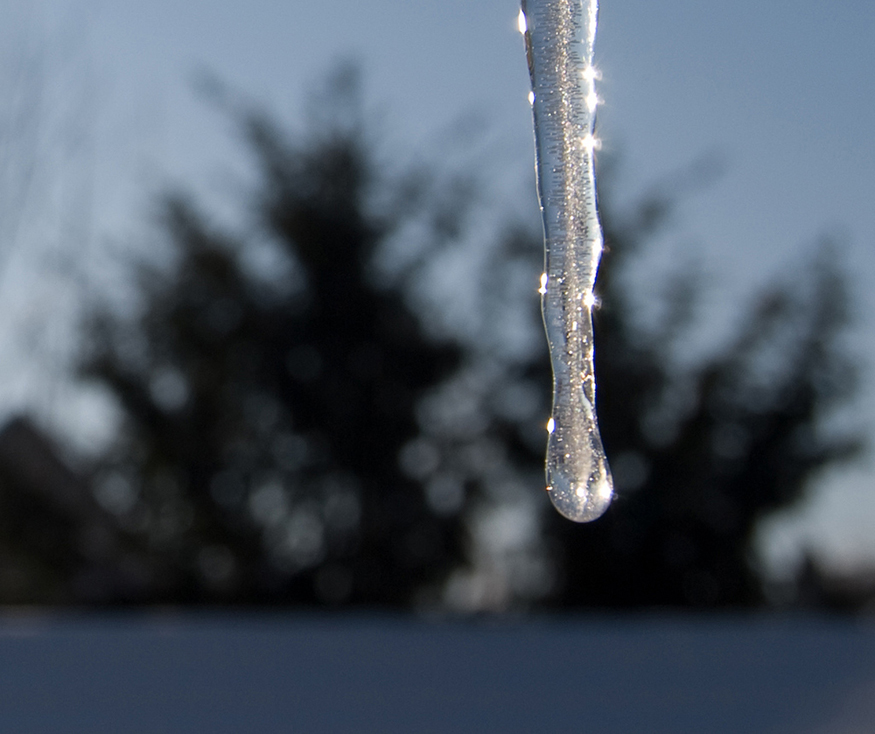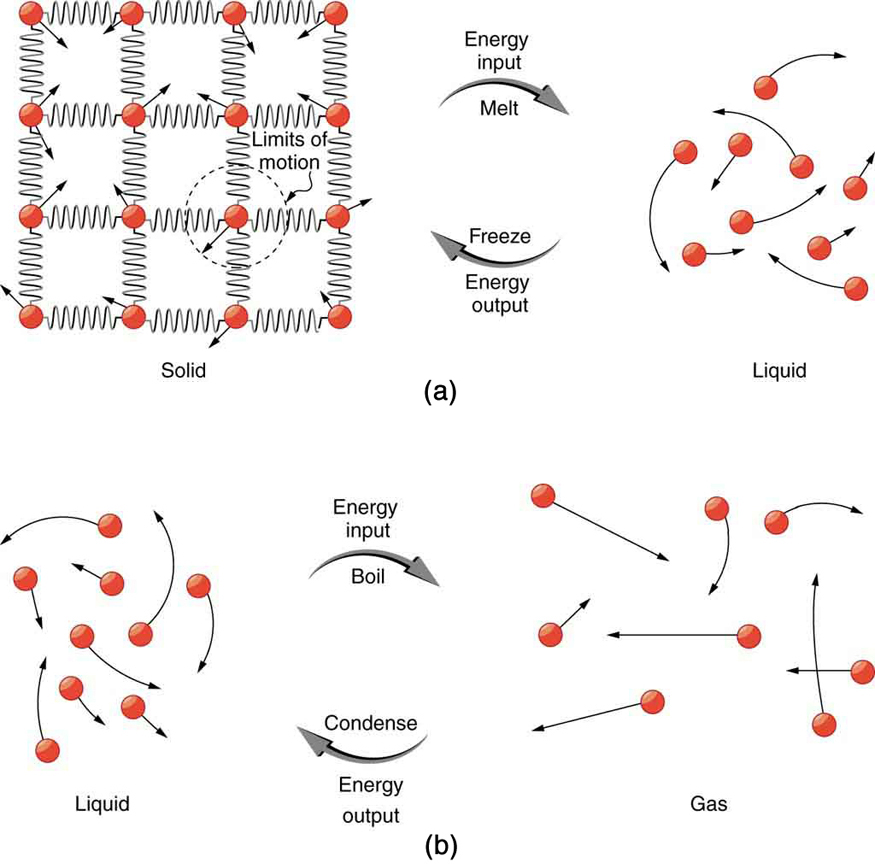# 14.3 Phase change and latent heat

 Page 1 / 9
• Examine heat transfer.
• Calculate final temperature from heat transfer.

So far we have discussed temperature change due to heat transfer. No temperature change occurs from heat transfer if ice melts and becomes liquid water (i.e., during a phase change). For example, consider water dripping from icicles melting on a roof warmed by the Sun. Conversely, water freezes in an ice tray cooled by lower-temperature surroundings.Heat from the air transfers to the ice causing it to melt. (credit: Mike Brand)

Energy is required to melt a solid because the cohesive bonds between the molecules in the solid must be broken apart such that, in the liquid, the molecules can move around at comparable kinetic energies; thus, there is no rise in temperature. Similarly, energy is needed to vaporize a liquid, because molecules in a liquid interact with each other via attractive forces. There is no temperature change until a phase change is complete. The temperature of a cup of soda initially at $0º\text{C}$ stays at $0º\text{C}$ until all the ice has melted. Conversely, energy is released during freezing and condensation, usually in the form of thermal energy. Work is done by cohesive forces when molecules are brought together. The corresponding energy must be given off (dissipated) to allow them to stay together [link] .

The energy involved in a phase change depends on two major factors: the number and strength of bonds or force pairs. The number of bonds is proportional to the number of molecules and thus to the mass of the sample. The strength of forces depends on the type of molecules. The heat $Q$ required to change the phase of a sample of mass $m$ is given by

where the latent heat of fusion, ${L}_{\text{f}}$ , and latent heat of vaporization, ${L}_{\text{v}}$ , are material constants that are determined experimentally. See ( [link] ).(a) Energy is required to partially overcome the attractive forces between molecules in a solid to form a liquid. That same energy must be removed for freezing to take place. (b) Molecules are separated by large distances when going from liquid to vapor, requiring significant energy to overcome molecular attraction. The same energy must be removed for condensation to take place. There is no temperature change until a phase change is complete.

Latent heat is measured in units of J/kg. Both ${L}_{\text{f}}$ and ${L}_{\text{v}}$ depend on the substance, particularly on the strength of its molecular forces as noted earlier. ${L}_{\text{f}}$ and ${L}_{\text{v}}$ are collectively called latent heat coefficients . They are latent , or hidden, because in phase changes, energy enters or leaves a system without causing a temperature change in the system; so, in effect, the energy is hidden. [link] lists representative values of ${L}_{\text{f}}$ and ${L}_{\text{v}}$ , together with melting and boiling points.

The table shows that significant amounts of energy are involved in phase changes. Let us look, for example, at how much energy is needed to melt a kilogram of ice at $0º\text{C}$ to produce a kilogram of water at $0°\text{C}$ . Using the equation for a change in temperature and the value for water from [link] , we find that $Q={\text{mL}}_{\text{f}}=\left(1\text{.}0\phantom{\rule{0.25em}{0ex}}\text{kg}\right)\left(\text{334}\phantom{\rule{0.25em}{0ex}}\text{kJ/kg}\right)=\text{334}\phantom{\rule{0.25em}{0ex}}\text{kJ}$ is the energy to melt a kilogram of ice. This is a lot of energy as it represents the same amount of energy needed to raise the temperature of 1 kg of liquid water from $0º\text{C}$ to $\text{79}\text{.}8º\text{C}$ . Even more energy is required to vaporize water; it would take 2256 kJ to change 1 kg of liquid water at the normal boiling point ( $\text{100º}\text{C}$ at atmospheric pressure) to steam (water vapor). This example shows that the energy for a phase change is enormous compared to energy associated with temperature changes without a phase change.

does the force in a system result in the energy transfer?
full meaning of GPS system
how to prove that Newton's law of universal gravitation F = GmM ______ R²
sir dose it apply to the human system
prove that the centrimental force Fc= M1V² _________ r
prove that centripetal force Fc = MV² ______ r
Kaka
how lesers can transmit information
griffts bridge derivative
below me
please explain; when a glass rod is rubbed with silk, it becomes positive and the silk becomes negative- yet both attracts dust. does dust have third types of charge that is attracted to both positive and negative
what is a conductor
Timothy
hello
Timothy
below me
why below you
Timothy
no....I said below me ...... nothing below .....ok?
dust particles contains both positive and negative charge particles
Mbutene
corona charge can verify
Stephen
when pressure increases the temperature remain what?
remains the temperature
betuel
what is frequency
define precision briefly
CT scanners do not detect details smaller than about 0.5 mm. Is this limitation due to the wavelength of x rays? Explain.
hope this helps
what's critical angle
The Critical Angle Derivation So the critical angle is defined as the angle of incidence that provides an angle of refraction of 90-degrees. Make particular note that the critical angle is an angle of incidence value. For the water-air boundary, the critical angle is 48.6-degrees.
okay whatever
Chidalu
pls who can give the definition of relative density?
Temiloluwa
the ratio of the density of a substance to the density of a standard, usually water for a liquid or solid, and air for a gas.
Chidalu
What is momentum
mass ×velocity
Chidalu
it is the product of mass ×velocity of an object
Chidalu
how do I highlight a sentence]p? I select the sentence but get options like copy or web search but no highlight. tks. src
then you can edit your work anyway you wantByBy OpenStaxBy OpenStaxBy OpenStaxBy Angelica LitoBy OpenStaxByBy Stephen VoronBy OpenStaxBy OpenStaxBy Richley Crapo